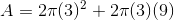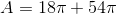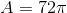## Example Questions

### Example Question #3 : How To Find The Volume Of A Cylinder

What is the surface area of a cylinder with a radius of 2 cm and a height of 10 cm?

32π cm2

48π cm2

40π cm2

36π cm2

56π cm2

48π cm2

Explanation:

SAcylinder = 2πrh + 2πr2 = 2π(2)(10) + 2π(2)2 = 40π + 8π = 48π cm2

### Example Question #122 : Solid Geometry

If a cylinder has a radius,, of 2 inches and a height,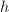, of 5 inches, what is the total surface area of the cylinder?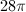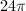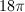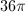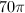Explanation:

The total surface area will be equal to the area of the two bases added to the area of the outer surface of the cylinder. If "unwrapped" the area of the outer surface is simply a rectangle with the height of the cylinder and a base equal to the circumference of the cylinder base. We can use these relationships to find a formula for the total area of the cylinder.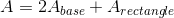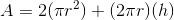Use the given radius and height to solve for the final area.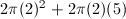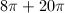### Example Question #124 : Solid Geometry

What is the surface area of a cylinder with a base diameter of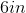and a height of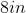?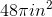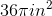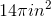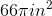Explanation:

Area of a circle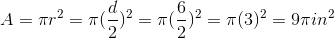Circumference of a circle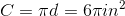Surface area of a cylinder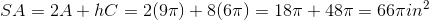### Example Question #1 : How To Find The Surface Area Of A Cylinder

What is the surface area of a cylinder with a radius ofand a height of? Give your answer in terms of.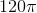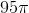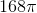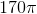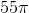Explanation:

To find the surface area of a cylinder with radiusand heightuse the equation: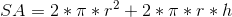Thus for a cylinder with a radius of 5 and a height of 7 we get:### Example Question #2 : How To Find The Surface Area Of A Cylinder

Find the surface area of a cylinder whose height is 6 and radius is 7.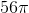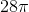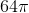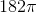Explanation:

To solve, simply use the formula for surface area of a cylinder.

First, identify all known information.

Height = 6

Substitute these values into the surface area equation and solve.

Thus,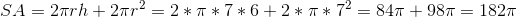### Example Question #3 : How To Find The Surface Area Of A Cylinder

A grain silo in the shape of a right circular cylinder is erected vertically, as shown below. The silo is then covered with corrugated steel. If the cylinder istall and has a circumference of, how much corrugated steel, in square meters, must be used to cover the visible portion of the silo?Explanation:

The surface area of a cylinder can be calculated using the following formula:In this equation, the variable,, is the radius of the base of the cylinder andis the height of the cylinder.

In this case, we must also remember not to include one of the two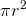measurements, since the bottom face that is in contact with the ground will not be covered with corrugated steel. We need to modify our surface area formula in the following way:We are given the circumference of the cylinder; therefore, we can use this information to solve for the radius.Since the circumference is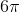, we know the radius is 3. Now we can insert these values to the modified surface area equation and solve for the coverable surface area of the silo.### Example Question #121 : Solid Geometry

The volume of a cylinder is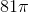. If the radius of the cylinder is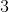, what is the surface area of the cylinder?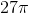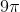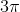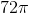Explanation:

The volume of a cylinder is equal to: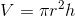Use this formula and the given radius to solve for the height.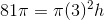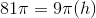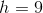Now that we know the height, we can solve for the surface area. The surface area of a cylinder is equal to the area of the two bases plus the area of the outer surface. The outer surface can be "unwrapped" to form a rectangle with a height equal to the cylinder height and a base equal to the circumference of the cylinder base. Add the areas of the two bases and this rectangle to find the total area.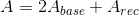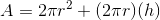Use the radius and height to solve.